# Difference between revisions of "Wronskian"

Wronski determinant

The determinant of a system ofvector-functions of dimension,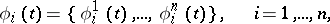(1)

of the typeThe Wronskian of a system ofscalar functions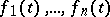(2)

which have derivatives up to order(inclusive) is the determinant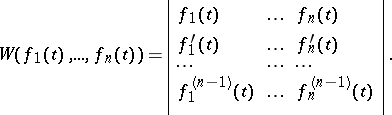(3)

The concept was first introduced by J. Wronski .

If the vector-functions (1) are linearly dependent on a set, thenIf the scalar functions (2) are linearly dependent on a set, thenThe converse theorems are usually not true: Identical vanishing of a Wronskian on some set is not a sufficient condition for linear dependence offunctions on this set.

Let the vector-functions (1) be the solutions of a linear homogeneous-th order system,, with an-dimensional matrixthat is continuous on an interval. If these solutions constitute a fundamental system, then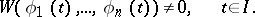If the Wronskian of these solutions is equal to zero in at least one point of, it is identically equal to zero on, and the functions (1) are linearly dependent. The Liouville formula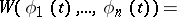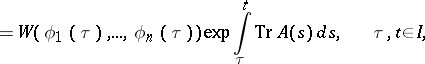where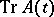is the trace of the matrix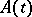, is applicable.

Let the functions (2) be the solutions of a linear homogeneous-th order equation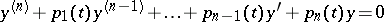with continuous coefficients on the interval. If these solutions constitute a fundamental system, then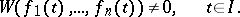If the Wronskian of these solutions is zero in at least one point of, it is identically equal to zero on, and the functions (2) are linearly dependent. The Liouville formula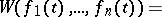applies.

How to Cite This Entry:
Wronskian. Encyclopedia of Mathematics. URL: http://encyclopediaofmath.org/index.php?title=Wronskian&oldid=17717
This article was adapted from an original article by N.Kh. Rozov (originator), which appeared in Encyclopedia of Mathematics - ISBN 1402006098. See original article